# Baby steps in Python – Exploratory analysis in Python (using Pandas)

In the last 2 posts of this series, we looked at how to install Python with iPython interface and several useful libraries and data structures, which are available in Python. If you have not gone through these posts and are new to Python, I would recommend that you go through the previous posts before going ahead.

In order to explore our data further, let me introduce you to another animal (as if Python was not enough!) – Pandas

Pandas are one of the most useful data analysis library in Python (I know these names sounds weird, but hang on!). They have been instrumental in increasing the use of Python in data science community. In this tutorial, we will use Pandas to read a data set from a Kaggle competition, perform exploratory analysis and build our first basic categorization algorithm for solving this problem.

### Introduction to Series and Dataframes

Series can be understood as a 1 dimensional labelled / indexed array. You can access individual elements of this series through these labels.

A dataframe is similar to Excel workbook – you have column names referring to columns and you have rows, which can be accessed with use of row numbers. The essential difference being that column names and row numbers are known as column and row index, in case of dataframes.

Series and dataframes form the core data model for Pandas in Python. The data sets are first read into these dataframes and then various operations (e.g. group by, aggregation etc.) can be applied very easily to its columns.

### Kaggle dataset – Titanic: Machine Learning from Disaster

You can download the dataset from Kaggle. Here is the description of variables as provided by Kaggle:

```VARIABLE DESCRIPTIONS:
survival        Survival
(0 = No; 1 = Yes)
pclass          Passenger Class
(1 = 1st; 2 = 2nd; 3 = 3rd)
name            Name
sex             Sex
age             Age
sibsp           Number of Siblings/Spouses Aboard
parch           Number of Parents/Children Aboard
ticket          Ticket Number
fare            Passenger Fare
cabin           Cabin
embarked        Port of Embarkation
(C = Cherbourg; Q = Queenstown; S = Southampton)

SPECIAL NOTES:
Pclass is a proxy for socio-economic status (SES)
1st ~ Upper; 2nd ~ Middle; 3rd ~ Lower

Age is in Years; Fractional if Age less than One (1)
If the Age is Estimated, it is in the form xx.5

With respect to the family relation variables (i.e. sibsp and parch)
some relations were ignored.  The following are the definitions used
for sibsp and parch.

Sibling:  Brother, Sister, Stepbrother, or Stepsister of Passenger Aboard Titanic
Spouse:   Husband or Wife of Passenger Aboard Titanic (Mistresses and Fiances Ignored)
Parent:   Mother or Father of Passenger Aboard Titanic
Child:    Son, Daughter, Stepson, or Stepdaughter of Passenger Aboard Titanic

Other family relatives excluded from this study include cousins,
nephews/nieces, aunts/uncles, and in-laws.  Some children travelled
only with a nanny, therefore parch=0 for them.  As well, some
travelled with very close friends or neighbors in a village, however,
the definitions do not support such relations.```

### Let the exploration begin

To begin, start iPython interface in Inline Pylab mode by typing following on your terminal / windows command prompt:

`ipython notebook --pylab=inline`

This opens up iPython notebook in pylab environment, which has a few useful libraries already imported. Also, you will be able to plot your data inline, which makes this a really good environment for interactive data analysis. You can check whether the environment has loaded correctly, by typing the following command (and getting the output as seen in the figure below):

`plot(arange(5))`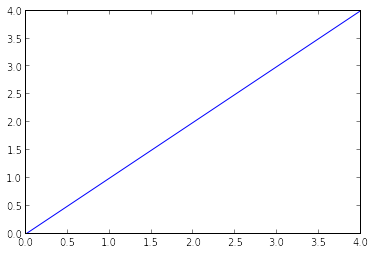I am currently working in Linux, and have stored the dataset in the following location:

/home/kunal/Downloads/kaggle/train.csv

#### Importing libraries and the data set:

Following are the libraries we will use during this tutorial:

• numpy
• matplotlib
• pandas

You can read a brief description about each of these libraries here. Please note that you do not need to import matplotlib and numpy because of Pylab environment. I have still kept them in the code, in case you use the code in a different environment.

After importing the library, you read the dataset using function read_csv(). This is how the code looks like till this stage:

```import pandas as pd
import numpy as np
import matplotlib as plt

df = pd.read_csv("/home/kunal/Downloads/kaggle/train.csv") #Reading the dataset in a dataframe using Pandas
```

#### Quick data exploration:

Once you have read the dataset, you can have a look at few top rows by using the function head()

`df.head(10)`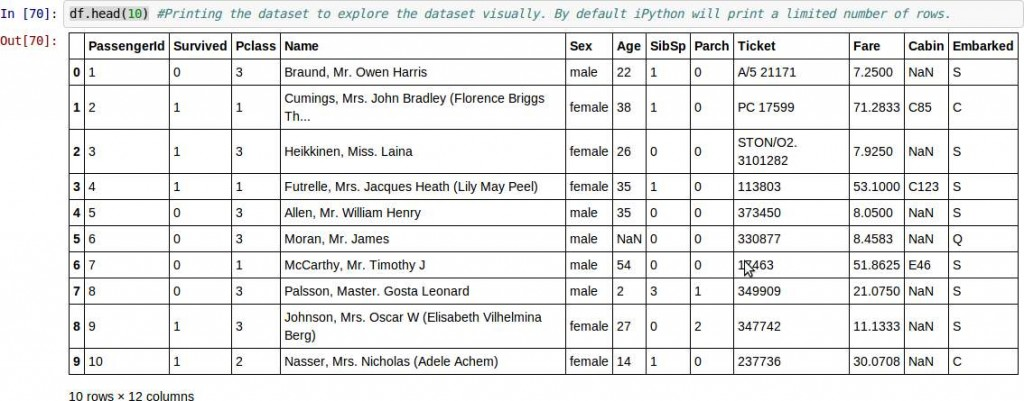This should print 10 rows. Alternately, you can also look at more rows by printing the dataset.

Next, you can look at summary of numerical fields by using describe() function

`df.describe()`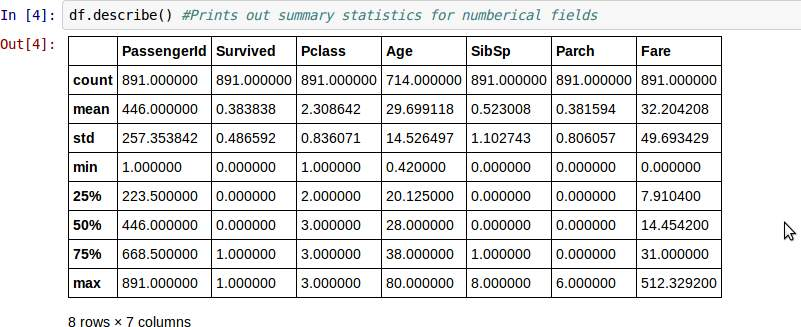describe() function would provide count, mean, standard deviation (std), min, quartiles and max in its output (Read this article to refresh basic statistics to understand population distribution)

Here are a few inferences, you can draw by looking at the output of describe() function:

1. Age has (891 – 714) 277 missing values.
2. We can also look that about 38% passangers survived the tragedy. How? The mean of survival field is 0.38 (Remember, survival has value 1 for those who survived and 0 otherwise)
3. By looking at percentiles of Pclass, you can see that more than 50% of passengers belong to class 3,
4. The age distribution seems to be in line with expectation. Same with SibSp and Parch
5. The fare seems to have values with 0 indicating possibility of some free tickets or data errors. On the other extreme, 512 looks like a possible outlier / error

In addition to these statistics, you can also look at the median of these variables and compare them with mean to see possible skew in the dataset. Median can be found out by:

`df['Age'].median()`

For the non-numerical values (e.g. Sex, Embarked etc.), we can look at unique values to understand whether they make sense or not. Since Name would be a free flowing field, we will exclude it from this analysis. Unique value can be printed by following command:

`df['Sex'].unique()`

Similarly, we can look at unique values of port of embarkment.

#### Distribution analysis:

Now that we are familiar with basic data characteristics, let us study distribution of various variables. Let us start with numeric variables – namely Age and Fare

We plot their histograms using the following commands:

```fig = plt.pyplot.figure()
ax = fig.add_subplot(111)
ax.hist(df['Age'], bins = 10, range = (df['Age'].min(),df['Age'].max()))
plt.pyplot.title('Age distribution')
plt.pyplot.xlabel('Age')
plt.pyplot.ylabel('Count of Passengers')
plt.pyplot.show()
```

and

```fig = plt.pyplot.figure()
ax = fig.add_subplot(111)
ax.hist(df['Fare'], bins = 10, range = (df['Fare'].min(),df['Fare'].max()))
plt.pyplot.title('Fare distribution')
plt.pyplot.xlabel('Fare')
plt.pyplot.ylabel('Count of Passengers')
plt.pyplot.show()
```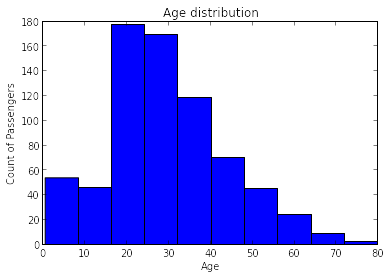Next, we look at box plots to understand the distributions. Box plot for fare can be plotted by:

`df.boxplot(column='Fare')`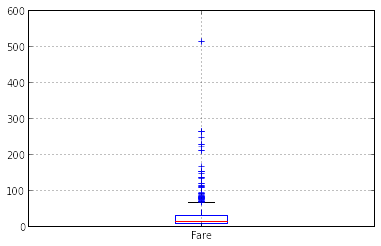This shows a lot of Outliers. Part of this can be driven by the fact that we are looking at fare across the 3 passenger classes. Let us segregate them by Passenger class:

`df.boxplot(column='Fare', by = 'Pclass')`Clearly, both Age and Fare require some amount of data munging. Age has about 31% missing values, while Fare has a few Outliers, which demand deeper understanding. We will take this up later (in the next tutorial).

#### Categorical variable analysis:

Now that we understand distributions for Age and Fare, let us understand categorical variables in more details. Following code plots the distribution of population by PClass and their probability of survival:

```temp1 = df.groupby('Pclass').Survived.count()
temp2 = df.groupby('Pclass').Survived.sum()/df.groupby('Pclass').Survived.count()
fig = plt.pyplot.figure(figsize=(8,4))
ax1 = fig.add_subplot(121)
ax1.set_xlabel('Pclass')
ax1.set_ylabel('Count of Passengers')
ax1.set_title("Passengers by Pclass")
temp1.plot(kind='bar')

ax2 = fig.add_subplot(122)
temp2.plot(kind = 'bar')
ax2.set_xlabel('Pclass')
ax2.set_ylabel('Probability of Survival')
ax2.set_title("Probability of survival by class")
```You can plot similar graphs by Sex and port of embarkment.

Alternately, these two plots can also be visualized by combining them in a stacked chart:

```temp3 = pd.crosstab([df.Pclass, df.Sex], df.Survived.astype(bool))
temp3.plot(kind='bar', stacked=True, color=['red','blue'], grid=False)```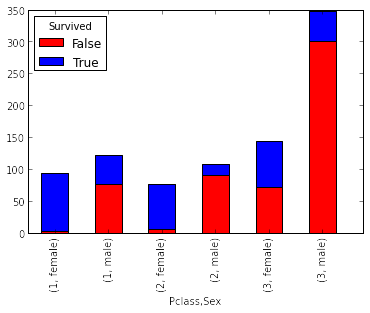You can also add port of embankment into the mix:If you have not realized already, we have just created two basic classification algorithms here, one based on Pclass and Sex, while other on 3 categorical variables (including port of embankment). You can quickly code this to create your first submission on Kaggle.

#### End Notes:

In this post, we saw how we can do exploratory analysis in Python using Pandas. I hope your love for pandas (the animal) would have increased by now – given the amount of help, the library can provide you in analyzing datasets.

We will start the next tutorial from this stage, where we will explore Age and Fare variables further, perform data munging and create a dataset for applying various modeling techniques. If you are following this series, we have covered a lot of ground in this tutorial. I would strongly urge that you take another dataset and problem and go through an independent example before we publish the next post in this series.

If you come across any difficulty while doing so, or you have any thoughts / suggestions / feedback on the post, please feel free to post them through comments below.

### If you like what you just read & want to continue your analytics learning, subscribe to our emails, follow us on twitter or like our facebook page.

You can also read this article on Analytics Vidhya's Android APPThis article is quite old and you might not get a prompt response from the author. We request you to post this comment on Analytics Vidhya's Discussion portal to get your queries resolved

### 15 Comments

•Gaurav says:

Thanks Kunal,

Its a wonderful article to get started with Data Analysis in Python.

I am getting empty plot for Age distribution. Is this because df[‘Age’].min() = 0.41999999999999998

•Kunal Jain says:

Gaurav,

Can you check if you are getting a histogram output with following command:

df[‘Age’].hist()

If you still get it empty, can you send the output of the df.describe() to me via email? Which version of Python, matplotlib and pandas are you using through which interface?

Regards,
Kunal

•Gaurav says:

Thanks Kunal,

When I run df[‘Age’].hist(), the histogram is correctly displayed. I am using Enthought canopy and IPython Notebook.

Regards
Gaurav

•Gaurav says:

The version of Python is:

2.7.6 | 64-bit | (default, Jun 4 2014, 16:30:34) [MSC v.1500 64 bit (AMD64)]

Version of Pandas is: 0.14.0

Version of Matplotlib is: 1.3.1

•Phil Renaud says:

Really excellent overview – thank you! Any recommendations for which data set to look at next?

•Kunal Jain says:

Thanks Phil! It entirely depends on your past experience. If you already know stats and predictive modeling technique – but are learning Python as a new tool – I would say that you should take up a bigger dataset and a more complex problem (e.g. Tree classification or movie review mining on Kaggle).

If you are learning both the tools and the techniques for the first time, I would say to take a few well documented problems – example Iris dataset, so that you can learn about various techniques and their results from internet.

Hope this helps.

Regards,
Kunal

•Stas says:

Good article! Thanks!

It’s better to avoid using of “–pylab=inline” – see details here: http://carreau.github.io/posts/10-No-PyLab-Thanks.ipynb.html

•Vasudev says:

Thanks Kunal for great article 🙂 . Could you please let me know how can I use help command in python? For example if I want to structure and paramenters of the function read_csv how can I do that? we can achieve that in R just by typing ?read.csv . is there any thing handy like this in python for quick understanding of parameters and structure?

Thanks,
Vasudev Daruuvuri

•Benjamin says:

Thanks for the article.
I have noticed a minor errata. The missing values for Age aren’t 277 but 177: (891 – 714)

•Kunal Jain says:

Thanks Benjamin for pointing it out. Will correct it.

Regards,
Kunal

•Rehan Singh says:

Excellent blog! Thanks! Python is a duck, and not a pelican or something of that sort, meaning that when you type a command, the object’s methods and properties determine the output, rather than being inherited from a particular class. Essentially, it doesn’t matter what type of object you’re using—if it can be done with that object, Python will do it. You can create your own classes with a structure that works for your data, and run regular Python syntax on them, which is helpful with large or unique datasets. You can also create your own dictionaries, basically making a cache of information to refer back to later in on in your notebook, rather than having to create it from scratch every time.
Python is a powerful, flexible, open-source language that is easy to learn, easy to use, and has powerful libraries for data manipulation and analysis. Its simple syntax is very accessible to programming novices, and will look familiar to anyone with experience in Matlab, C/C++, Java, or Visual Basic. Python has a unique combination of being both a capable general-purpose programming language as well as being easy to use for analytical and quantitative computing.

•Shane Keller says:

Thanks for this tutorial Kunal. I found it to be a great balance of technically informative yet easy to understand. I think the baby steps idea is working ;).

•MichaelReinhar9 says:

Thanks, Kunal, for this wonderful tutorial and the whole site. I think it is one of the most useful over all on the web.

I am just working through the tutorial now and I have run into a problem I hope you can help me with. On the first chart where we see the two bar charts side by side, one showing the counts of passengers by class and the other, on the right, showing the probability of survival by class: I am having a bit of trouble really understanding the code.

The first line is defines temp1 by ‘df.groupby(‘Pclass’).survived.count()’. I don’t see why ‘Survived’ is in there? If we just want the count of people in the class wouldn’t we just get the counts by class? If so, it would seem sufficient to just have df.groupby(‘Pclass’).count(), no?

Of course I tried that and it didn’t work. In fact the both of the two panels went blank and a couple of really weird graphs showed up underneath.

I know this tutorial has been up for over a year so don’t worry if you don’t answer. I am going to work the other tutorials and if I find the answer to this myself I will post the answer. I have a feeling that since no one else had the question I have that the question is answered in another tutorial.

In any case, thanks for these kick-ass tutorials!

•MichaelReinhar9 says:

Ok, found the answer to my own dumb question. The ‘.survived’ is just there to give the count() method something to work on. It just has to be a variable (a column) that is complete. It actually works the same if you use .passengerId for the first panel to get the counts by Pclass, though in the right hand panel you need Survived to get the probability of survival.

•Schiff says:

your website is a great resource to start learning data science. thank you for sharing!Download ResourceDownload Resource